# Passive Integrator and Differentiator Circuits

## AC Electric Circuits

• #### Question 1

Calculus is a branch of mathematics that originated with scientific questions concerning rates of change. The easiest rates of change for most people to understand are those dealing with time. For example, a student watching their savings account dwindle over time as they pay for tuition and other expenses is very concerned with rates of change (dollars per year being spent).

In calculus, we have a special word to describe rates of change: derivative. One of the notations used to express a derivative (rate of change) appears as a fraction. For example, if the variable S represents the amount of money in the student’s savings account and t represents time, the rate of change of dollars over time would be written like this:

 dS dt

The following set of figures puts actual numbers to this hypothetical scenario:

Date: November 20
Saving account balance (S) = \$12,527.33
Rate of spending ([dS/dt]) = -5,749.01 per year

List some of the equations you have seen in your study of electronics containing derivatives, and explain how rate of change relates to the real-life phenomena described by those equations.

• #### Question 2

How does the rate of charge flow (current) into and out of a capacitor relate to the amount of voltage across its terminals? How does the rate of water flow into and out of a vessel relate to the amount of water stored in that vessel?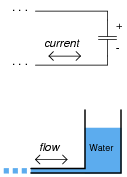• #### Question 3

According to the “Ohm’s Law” formula for a capacitor, capacitor current is proportional to the time-derivative of capacitor voltage:

 i = C dv dt

Another way of saying this is to state that the capacitors differentiate voltage with respect to time, and express this time-derivative of voltage as a current.

Suppose we had an oscilloscope capable of directly measuring current, or at least a current-to-voltage converter that we could attach to one of the probe inputs to allow direct measurement of current on one channel. With such an instrument set-up, we could directly plot capacitor voltage and capacitor current together on the same display: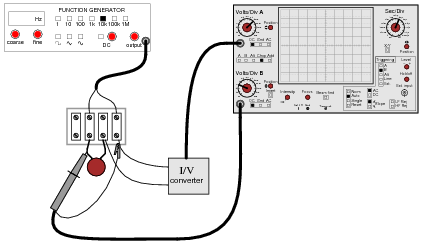For each of the following voltage waveforms (channel B), plot the corresponding capacitor current waveform (channel A) as it would appear on the oscilloscope screen: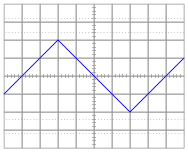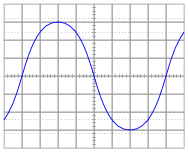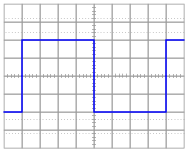Note: the amplitude of your current plots is arbitrary. What I’m interested in here is the shape of each current waveform!

• #### Question 4

According to the “Ohm’s Law” formula for a capacitor, capacitor current is proportional to the time-derivative of capacitor voltage:

 i = C dv dt

Another way of saying this is to state that the capacitors differentiate voltage with respect to time, and express this time-derivative of voltage as a current.

We may build a simple circuit to produce an output voltage proportional to the current through a capacitor, like this: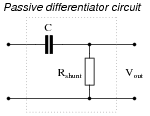The resistor is called a shunt because it is designed to produce a voltage proportional to current, for the purpose of a parallel (“shunt”)-connected voltmeter or oscilloscope to measure that current. Ideally, the shunt resistor is there only to help us measure current, and not to impede current through the capacitor. In other words, its value in ohms should be very small compared to the reactance of the capacitor (Rshunt << X

C).

Suppose that we connect AC voltage sources with the following wave-shapes to the input of this passive differentiator circuit. Sketch the ideal (time-derivative) output waveform shape on each oscilloscope screen, as well as the shape of the actual circuit’s output voltage (which will be non-ideal, of course):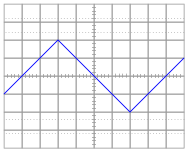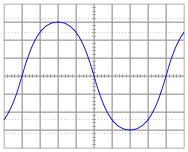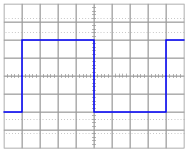Note: the amplitude of your plots is arbitrary. What I’m interested in here is the shape of the ideal and actual output voltage waveforms!

Hint: I strongly recommend building this circuit and testing it with triangle, sine, and square-wave input voltage signals to obtain the corresponding actual output voltage wave-shapes!

• #### Question 5

Generally speaking, how many “time constants” worth of time does it take for the voltage and current to “settle” into their final values in an RC or LR circuit, from the time the switch is closed?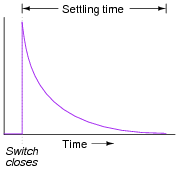• #### Question 6

Suppose a fellow electronics technician approaches you with a design problem. He needs a simple circuit that outputs brief pulses of voltage every time a switch is actuated, so that a computer receives a single pulse signal every time the switch is actuated, rather than a continuous “on” signal for as long as the switch is actuated: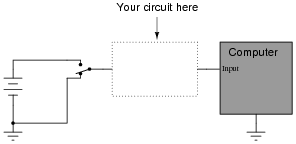The technician suggests you build a passive differentiator circuit for his application. You have never heard of this circuit before, but you probably know where you can research to find out what it is! He tells you it is perfectly okay if the circuit generates negative voltage pulses when the switch is de-actuated: all he cares about is a single positive voltage pulse to the computer each time the switch actuates. Also, the pulse needs to be very short: no longer than 2 milliseconds in duration.

Given this information, draw a schematic diagram for a practical passive differentiator circuit within the dotted lines, complete with component values.

• #### Question 7

Plot the output waveform of a passive differentiator circuit, assuming the input is a symmetrical square wave and the circuit’s RC time constant is about one-fifth of the square wave’s pulse width: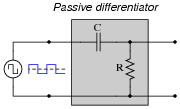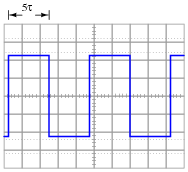• #### Question 8

Potentiometers are very useful devices in the field of robotics, because they allow us to represent the position of a machine part in terms of a voltage. In this particular case, a potentiometer mechanically linked to the joint of a robotic arm represents that arm’s angular position by outputting a corresponding voltage signal: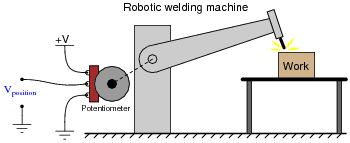As the robotic arm rotates up and down, the potentiometer wire moves along the resistive strip inside, producing a voltage directly proportional to the arm’s position. A voltmeter connected between the potentiometer wiper and ground will then indicate arm position. A computer with an analog input port connected to the same points will be able to measure, record, and (if also connected to the arm’s motor drive circuits) control the arm’s position.

If we connect the potentiometer’s output to a differentiator circuit, we will obtain another signal representing something else about the robotic arm’s action. What physical variable does the differentiator output signal represent?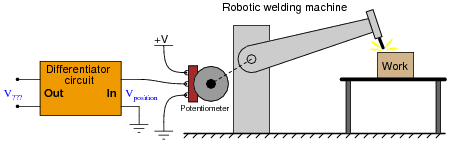• #### Question 9

One of the fundamental principles of calculus is a process called integration. This principle is important to understand because it is manifested in the behavior of capacitance. Thankfully, there are more familiar physical systems which also manifest the process of integration, making it easier to comprehend.

If we introduce a constant flow of water into a cylindrical tank with water, the water level inside that tank will rise at a constant rate over time: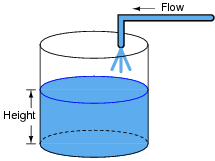In calculus terms, we would say that the tank integrates water flow into water height. That is, one quantity (flow) dictates the rate-of-change over time of another quantity (height).

Like the water tank, electrical capacitance also exhibits the phenomenon of integration with respect to time. Which electrical quantity (voltage or current) dictates the rate-of-change over time of which other quantity (voltage or current) in a capacitance? Or, to re-phrase the question, which quantity (voltage or current), when maintained at a constant value, results in which other quantity (current or voltage) steadily ramping either up or down over time?

• #### Question 10

One of the fundamental principles of calculus is a process called integration. This principle is important to understand because it is manifested in the behavior of inductance. Thankfully, there are more familiar physical systems which also manifest the process of integration, making it easier to comprehend.

If we introduce a constant flow of water into a cylindrical tank with water, the water level inside that tank will rise at a constant rate over time:In calculus terms, we would say that the tank integrates water flow into water height. That is, one quantity (flow) dictates the rate-of-change over time of another quantity (height).

Like the water tank, electrical inductance also exhibits the phenomenon of integration with respect to time. Which electrical quantity (voltage or current) dictates the rate-of-change over time of which other quantity (voltage or current) in an inductance? Or, to re-phrase the question, which quantity (voltage or current), when maintained at a constant value, results in which other quantity (current or voltage) steadily ramping either up or down over time?

• #### Question 11

Describe what happens to the capacitor voltage in this circuit over time as it is charged by the constant-current source: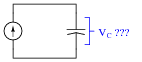Now, determine the ideal values for V and R that will result in similar behavior in a capacitor circuit powered by a voltage source rather than a current source:Your answers will, of course, be qualitative rather than quantitative. Explain whether or not the time constant for the voltage-source-powered circuit ought to be large or small, and why.

• #### Question 12

It is relatively easy to design and build an electronic circuit to make square-wave voltage signals. More difficult to engineer is a circuit that directly generates triangle-wave signals. A common approach in electronic design when triangle waves are needed for an application is to connect a passive integrator circuit to the output of a square-wave oscillator, like this:Anyone familiar with RC circuits will realize, however, that a passive integrator will not output a true triangle wave, but rather it will output a waveshape with “rounded” leading and trailing edges: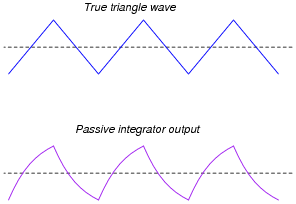What can be done with the values of R and C to best approximate a true triangle wave? What variable must be compromised to achieve the most linear edges on the integrator output waveform? Explain why this is so.

• #### Question 13

Design a passive integrator circuit using a resistor and inductor rather than a resistor and capacitor: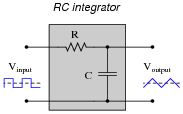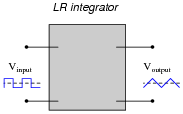In addition to completing the inductor circuit schematic, qualitatively state the preferred values of L and R to achieve an output waveform most resembling a true triangle wave. In other words, are we looking for a large or small inductor; a large or small resistor?

• #### Question 14

When you look at the schematic diagram for a passive integrator circuit, it ought to remind you of another type of circuit you’ve seen before: a passive filter circuit: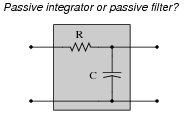What specific type of passive filter does a passive integrator circuit resemble? Is the resemblance the same for LR integrators as well, or just RC integrators? What does this resemblance tell you about the frequency response of a passive integrator circuit?

• #### Question 15

A “cheap” way to electronically produce waveforms resembling sine waves is to use a pair of passive integrator circuits, one to convert square waves into pseudo-triangle waves, and the next to convert pseudo-triangle waves into pseudo-sine waves: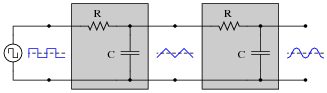From Fourier’s theory, we know that a square wave is nothing more than a series of sinusoidal waveforms: the fundamental frequency plus all odd harmonics at diminishing amplitudes. Looking at the two integrators as passive filter circuits, explain how it is possible to get a pseudo-sine wave from a square wave input as shown in the above diagram. Also, explain why the final output is not a true sine wave, but only resembles a sine wave.

• #### Question 16

Complete the following sentences with one of these phrases: “shorter than,” “longer than,” or “equal to”. Then, explain why the time constant of each circuit type must be so.

Passive integrator circuits should have time constants that are (fill-in-the-blank) the period of the waveform being integrated.
Passive differentiator circuits should have time constants that are (fill-in-the-blank) the period of the waveform being differentiated.
• #### Question 17

Both the input and the output of this circuit are square waves, although the output waveform is slightly distorted and also has much less amplitude: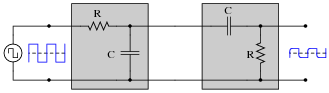You recognize one of the RC networks as a passive integrator, and the other as a passive differentiator. What does the likeness of the output waveform compared to the input waveform indicate to you about differentiation and integration as functions applied to waveforms?

• #### Question 18

Determine what the response will be to a constant DC voltage applied at the input of these (ideal) circuits: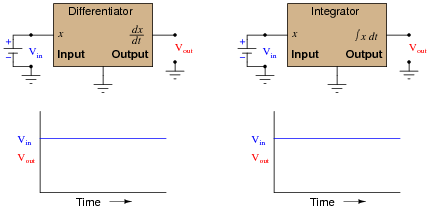• #### Question 19

In calculus, differentiation is the inverse operation of something else called integration. That is to say, differentiation “un-does” integration to arrive back at the original function (or signal). To illustrate this electronically, we may connect a differentiator circuit to the output of an integrator circuit and (ideally) get the exact same signal out that we put in: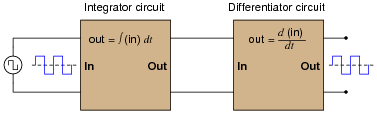Based on what you know about differentiation and differentiator circuits, what must the signal look like in between the integrator and differentiator circuits to produce a final square-wave output? In other words, if we were to connect an oscilloscope in between these two circuits, what sort of signal would it show us?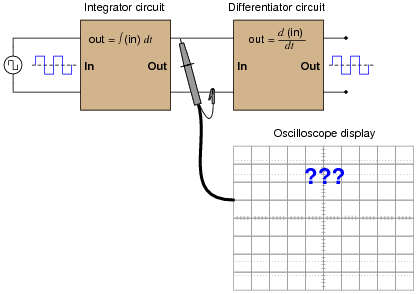• #### Question 20

When a circuit designer needs a circuit to provide a time delay, he or she almost always chooses an RC circuit instead of an LR circuit. Explain why this is.

• #### Question 21

An LR differentiator circuit is used to convert a triangle wave into a square wave. One day after years of proper operation, the circuit fails. Instead of outputting a square wave, it outputs a triangle wave, just the same as the waveform measured at the circuit’s input. Determine what the most likely component failure is in the circuit.

• #### Question 22

Calculate the output voltage of this passive differentiator circuit 1 millisecond after the rising edge of each positive square wave pulse (where the square wave transitions from -5 volts to 5 volts):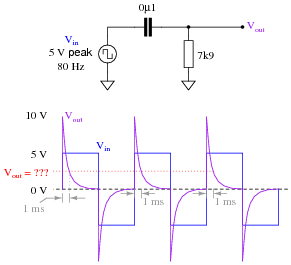• #### Question 23

Calculate the output voltage of this passive differentiator circuit 150 microseconds after the rising edge of each “clock” pulse (where the square wave transitions from 0 volts to 5 volts):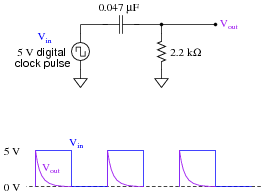• #### Question 24

A passive differentiator is used to “shorten” the pulse width of a square wave by sending the differentiated signal to a “level detector” circuit, which outputs a “high” signal ( 5 volts) whenever the input exceeds 3.5 volts and a “low” signal (0 volts) whenever the input drops below 3.5 volts: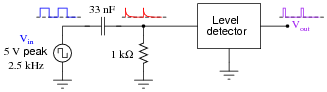Each time the differentiator’s output voltage signal spikes up to 5 volts and quickly decays to 0 volts, it causes the level detector circuit to output a narrow voltage pulse, which is what we want.

Calculate how wide this final output pulse will be if the input (square wave) frequency is 2.5 kHz.

• #### Question 25

A passive integrator circuit is energized by a square wave signal with a peak-to-peak amplitude of 12 volts and a frequency of 65.79 Hz: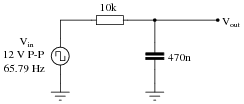Determine the peak-to-peak voltage of the output waveform: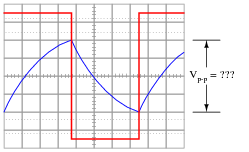Hint: the output waveform will be centered exactly half-way between the two peaks of the input square wave as shown in the oscilloscope image. Do not base your answer on relative sizes of the two waveforms, as I have purposely skewed the calibration of the oscilloscope screen image so the two waveforms are not to scale with each other.